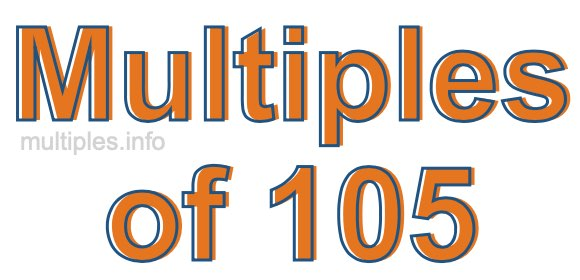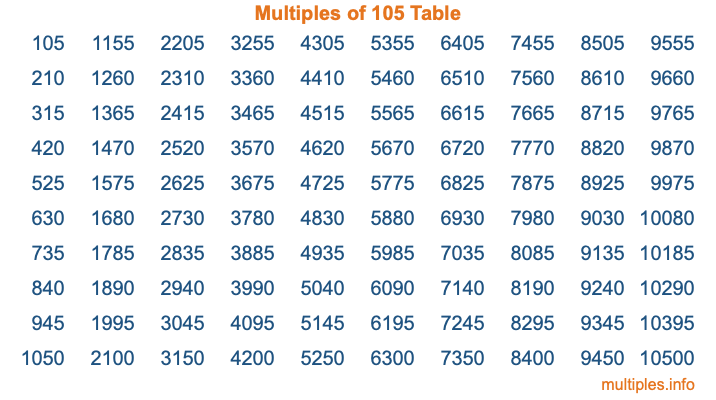Multiples of 105Welcome to the Multiples of 105 page. Here we will first teach you everything you will ever need to know about the multiples of 105, and then give you a study guide summary of everything we taught you to make sure you remember it all. Use this page to look up facts and learn information about the multiples of 105. This page will make you a multiples of one hundred five expert!

Definition of Multiples of 105
Multiples of 105 are all the numbers that when divided by 105 equal an integer. Each of the multiples of 105 are called a multiple. A multiple of 105 is created by multiplying 105 by an integer.

Therefore, to create a list of multiples of 105, you start with 1 multiplied by 105, then 2 multiplied by 105, then 3 multiplied by 105, and so on for as long as you want. Thus, the list of the first five multiples of 105 is 105, 210, 315, 420, and 525. To see a larger list of multiples of 105, see the printable image of Multiples of 105 further down on this page. We also have a category where you can choose any nth multiple of 105.

Multiples of 105 Checker
The Multiples of 105 Checker below checks to see if any number of your choice is a multiple of 105. In other words, it checks to see if there is any number (integer) that when multiplied by 105 will equal your number. To do that, we divide your number by 105. If the the quotient is an integer, then your number is a multiple of 105.

Is  a multiple of 105?

Least Common Multiple of 105 and ...
A Least Common Multiple (LCM) is the lowest multiple that two or more numbers have in common. This is also called the smallest common multiple or lowest common multiple and is useful to know when you are adding our subtracting fractions. Enter one or more numbers below (105 is already entered) to find the LCM.

Check out our LCM Calculator if you need more details about the Least Common Multiple or if you need the LCM for different numbers for adding and subtraction fractions.

nth Multiple of 105
As we stated above, 105 is the first multiple of 105, 210 is the second multiple of 105, 315 is the third multiple of 105, and so on. Enter a number below to find the nth multiple of 105.

th multiple of 105

Multiples of 105 vs Factors of 105
105 is a multiple of 105 and a factor of 105, but that is where the similarities end. All postive multiples of 105 are 105 or greater than 105. All positive factors of 105 are 105 or less than 105.

Below is the beginning list of multiples of 105 and the factors of 105 so you can compare:

Multiples of 105: 105, 210, 315, 420, 525, etc.

Factors of 105: 1, 3, 5, 7, 15, 21, 35, 105

As you can see, the multiples of 105 are all the numbers that you can divide by 105 to get a whole number. The factors of 105, on the other hand, are all the whole numbers that you can multiply by another whole number to get 105.

It's also interesting to note that if a number (x) is a factor of 105, then 105 will also be a multiple of that number (x).

Multiples of 105 vs Divisors of 105
The divisors of 105 are all the integers that 105 can be divided by evenly. Below is a list of the divisors of 105.

Divisors of 105: 1, 3, 5, 7, 15, 21, 35, 105

The interesting thing to note here is that if you take any multiple of 105 and divide it by a divisor of 105, you will see that the quotient is an integer.

Multiples of 105 Table
Below is an image of the first 100 multiples of 105 in a table. The table is in chronological order, column by column. The first column has the first ten multiples of 105, the second column has the next ten multiples of 105, and so on.The Multiples of 105 Table is also referred to as the 105 Times Table or Times Table of 105. You are welcome to print out our table for your studies.

Negative Multiples of 105
Although not often discussed or needed in math, it is worth mentioning that you can make a list of negative multiples of 105 by multiplying 105 by -1, then by -2, then by -3, and so on, to get the following list of negative multiples of 105:

-105, -210, -315, -420, -525, etc.

Multiples of 105 Summary
Below is a summary of important Multiples of 105 facts that we have discussed on this page. To retain the knowledge on this page, we recommend that you read through the summary and explain to yourself or a study partner why they hold true.

There are an infinite number of multiples of 105.

A multiple of 105 divided by 105 will equal a whole number.

105 divided by a factor of 105 equals a divisor of 105.

The nth multiple of 105 is n times 105.

The largest factor of 105 is equal to the first positive multiple of 105.

105 is a multiple of every factor of 105.

105 is a multiple of 105.

A multiple of 105 divided by a divisor of 105 equals an integer.

105 divided by a divisor of 105 equals a factor of 105.

Any integer times 105 will equal a multiple of 105.

Multiples of a Number
Here you can get the multiples of another number, all with the same attention to detail as we did for multiples of 105 on this page.

Multiples of
Multiples of 106
Did you find our page about multiples of one hundred five educational? Do you want more knowledge? Check out the multiples of the next number on our list!